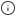# timescaledb_information.dimensions

Returns information about the dimensions of a hypertable. Hypertables can be partitioned on a range of different dimensions. By default, all hypertables are partitioned on time, but it is also possible to partition on other dimensions in addition to time.

For hypertables that are partitioned solely on time, `timescaledb_information.dimensions` returns a single row of metadata. For hypertables that are partitioned on more than one dimension, the call returns a row for each dimension.

For time-based dimensions, the metadata returned indicates the integer datatype, such as BIGINT, INTEGER, or SMALLINT, and the time-related datatype, such as TIMESTAMPTZ, TIMESTAMP, or DATE. For space-based dimension, the metadata returned specifies the number of `num_partitions`.

If the hypertable uses time data types, the `time_interval` column is defined. Alternatively, if the hypertable uses integer data types, the `integer_interval` and `integer_now_func` columns are defined.

## Available columns

NameTypeDescription
`hypertable_schema`TEXTSchema name of the hypertable
`hypertable_name`TEXTTable name of the hypertable
`dimension_number`BIGINTDimension number of the hypertable, starting from 1
`column_name`TEXTName of the column used to create this dimension
`column_type`REGTYPEType of the column used to create this dimension
`dimension_type`TEXTIs this a time based or space based dimension
`time_interval`INTERVALTime interval for primary dimension if the column type is a time datatype
`integer_interval`BIGINTInteger interval for primary dimension if the column type is an integer datatype
`integer_now_func`TEXT`integer_now`` function for primary dimension if the column type is an integer datatype
`num_partitions`SMALLINTNumber of partitions for the dimension###### Note

The `time_interval` and `integer_interval` columns are not applicable for space based dimensions.

## Sample usage

Get information about the dimensions of hypertables.

```--Create a time and space partitioned hypertableCREATE TABLE dist_table(time timestamptz, device int, temp float);SELECT create_hypertable('dist_table', 'time',  'device', chunk_time_interval=> INTERVAL '7 days', number_partitions=>3);
SELECT * from timescaledb_information.dimensions  ORDER BY hypertable_name, dimension_number;
-[ RECORD 1 ]-----+-------------------------hypertable_schema | publichypertable_name   | dist_tabledimension_number  | 1column_name       | timecolumn_type       | timestamp with time zonedimension_type    | Timetime_interval     | 7 daysinteger_interval  |integer_now_func  |num_partitions    |-[ RECORD 2 ]-----+-------------------------hypertable_schema | publichypertable_name   | dist_tabledimension_number  | 2column_name       | devicecolumn_type       | integerdimension_type    | Spacetime_interval     |integer_interval  |integer_now_func  |num_partitions    | 2```

Get information about dimensions of a hypertable that has two time-based dimensions.

```CREATE TABLE hyper_2dim (a_col date, b_col timestamp, c_col integer);SELECT table_name from create_hypertable('hyper_2dim', 'a_col');SELECT add_dimension('hyper_2dim', 'b_col', chunk_time_interval=> '7 days');
SELECT * FROM timescaledb_information.dimensions WHERE hypertable_name = 'hyper_2dim';
-[ RECORD 1 ]-----+----------------------------hypertable_schema | publichypertable_name   | hyper_2dimdimension_number  | 1column_name       | a_colcolumn_type       | datedimension_type    | Timetime_interval     | 7 daysinteger_interval  |integer_now_func  |num_partitions    |-[ RECORD 2 ]-----+----------------------------hypertable_schema | publichypertable_name   | hyper_2dimdimension_number  | 2column_name       | b_colcolumn_type       | timestamp without time zonedimension_type    | Timetime_interval     | 7 daysinteger_interval  |integer_now_func  |num_partitions    |```

Keywords